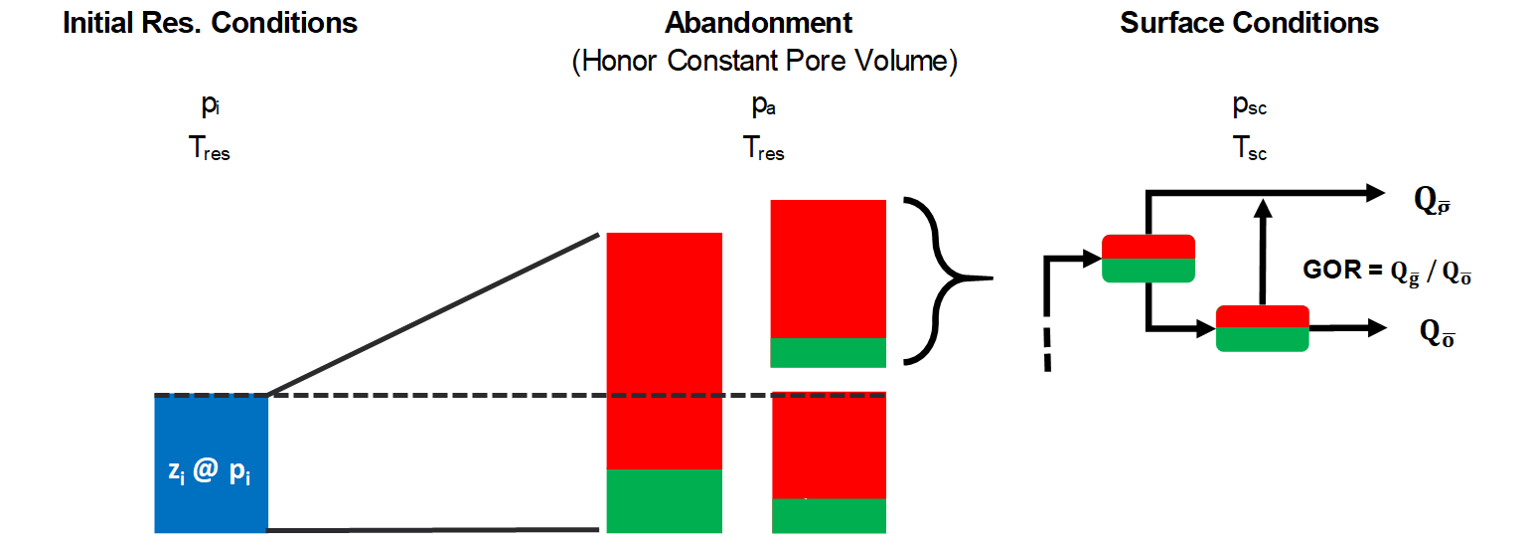# Simple Material BalanceIn this module, recovery factors for both oil ($$RF_{oil}$$) and gas ($$RF_{gas}$$) can be calculated with readily available data. The data required for calculating recovery factors is:

• Fluid definition
• Initial reservoir fluid composition (generated with any of the available methods)
• Surface process associated to the well (used to generate the black oil table)
• Initial reservoir pressure
• Reservoir temperature
• Abandonment pressure ($$p_{a}$$)
• Cumulative produced GOR ($$\frac{G_p}{N_p}$$)
How is cumulative produced GOR ($$\frac{G_p}{N_p}$$) estimated?

From type curves, numerical simulation or guessing.

## Recovery Factors

Recovery factor for oil ($$RF_{oil}$$) is defined as produced surface volume of oil (N) divided by original oil in place (N), i.e. $$RF_{oil} = \frac{N_p}{N}$$. Recovery factor for gas $$RF_{gas}$$ is defined as produced surface volume of gas (G) divided by original gas in place (G), i.e. $$RF_{gas} = \frac{G_p}{G}$$.